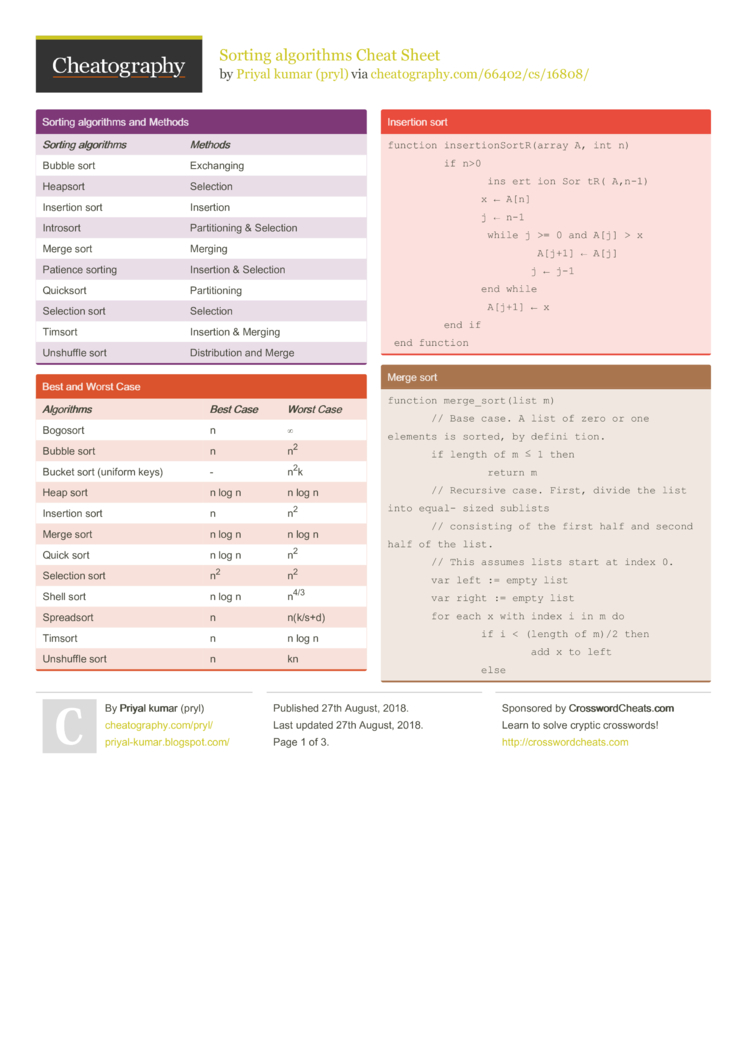# Sorting algorithms Cheat Sheet by pryl

algorithms of some sorting algorithms

### Sorting algorithms and Methods

 Sorting algori­thms Meth­ods Bubble sort Exchanging Heapsort Selection Insertion sort Insertion Introsort Partit­ioning & Selection Merge sort Merging Patience sorting Insertion & Selection Quicksort Partit­ioning Selection sort Selection Timsort Insertion & Merging Unshuffle sort Distri­bution and Merge

### Best and Worst Case

 Algo­rithms Best Case Worst Case Bogosort n ∞ Bubble sort n n2 Bucket sort (uniform keys) - n2k Heap sort n log n n log n Insertion sort n n2 Merge sort n log n n log n Quick sort n log n n2 Selection sort n2 n2 Shell sort n log n n4/3 Spreadsort n n(k/s+d) Timsort n n log n Unshuffle sort n kn

### Insertion sort

 ``` function insertionSortR(array A, int n)      if n>0         insertionSortR(A,n-1)         x ← A[n]         j ← n-1         while j >= 0 and A[j] > x             A[j+1] ← A[j]             j ← j-1         end while         A[j+1] ← x      end if  end function```

### Merge sort

 ```function merge_sort(list m)     // Base case. A list of zero or one elements is sorted, by definition.     if length of m ≤ 1 then         return m     // Recursive case. First, divide the list into equal-sized sublists     // consisting of the first half and second half of the list.     // This assumes lists start at index 0.     var left := empty list     var right := empty list     for each x with index i in m do         if i < (length of m)/2 then             add x to left         else             add x to right     // Recursively sort both sublists.     left := merge_sort(left)     right := merge_sort(right)     // Then merge the now-sorted sublists.     return merge(left, right)```

### Bogosort

 ```while not isInOrder(deck):     shuffle(deck)```

### Bucket sort

 ```function bucketSort(array, n) is   buckets ← new array of n empty lists   for i = 0 to (length(array)-1) do     insert array[i] into buckets[msbits(array[i], k)]   for i = 0 to n - 1 do     nextSort(buckets[i]);   return the concatenation of buckets, ...., buckets[n-1]```

### Sorting algorithm comple­xities

 Algo­rithms Average Case Memory comple­xity Bitonic sorter log2 n n log2 n Bogosort n × n! 1 Bubble sort n2 1 Bucket sort (uniform keys) n+k nk Burstsort n(k/d) n(k/d) Counting sort n+r n+r Heap sort n log n 1 Insertion sort n2 1 Introsort n log n log n LSD Radix Sort n(k/d) n+2d Merge sort n log n n MSD Radix Sort (in-place) n(k/d) 2d Patience sort - n Pigeonhole sort n+2k 2k Quicksort n log n log n Selection sort n2 1 Shell sort Depends on gap sequence 1 Spaghetti sort n n2 Spreadsort n(k/d) (k/d)2d Stooge sort n(log 3/log1.5) n Timsort n log n n

### Bubble sort

 ```procedure bubbleSort( A : list of sortable items )     n = length(A)     repeat         swapped = false         for i = 1 to n-1 inclusive do             if A[i-1] > A[i] then                 swap(A[i-1], A[i])                 swapped = true             end if         end for         n = n - 1     until not swapped end procedure```

### Quicksort

 ```algorithm quicksort(A, lo, hi) is     if lo < hi then         p := partition(A, lo, hi)         quicksort(A, lo, p - 1 )         quicksort(A, p + 1, hi) algorithm partition(A, lo, hi) is     pivot := A[hi]     i := lo     for j := lo to hi - 1 do         if A[j] < pivot then             swap A[i] with A[j]             i := i + 1     swap A[i] with A[hi]     return i```

### Selection sort

 ```procedure selection sort    list : array of items    n : size of list    for i = 1 to n - 1    / set current element as minimum/       min = i          / check the element to be minimum /       for j = i+1 to n          if list[j] < list[min] then             min = j;          end if       end for       / swap the minimum element with the current element/       if indexMin != i then          swap list[min] and list[i]       end if    end for end procedure```2 Pages
//media.cheatography.com/storage/thumb/pryl_sorting-algorithms.750.jpg

PDF (recommended)